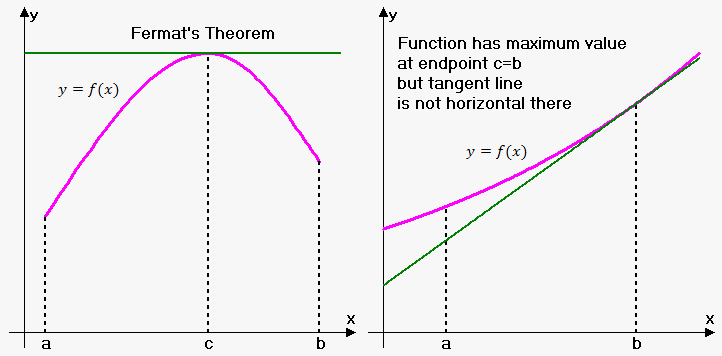# Fermat's Theorem

Knowing derivative of function ${f{{\left({x}\right)}}}$ allows us to make some conclusions about behavior of function ${y}={f{{\left({x}\right)}}}$.

Fermat's Theorem. Suppose function $y={f{{\left({x}\right)}}}$ is defined on interval ${\left[{a},{b}\right]}$ and at some point ${c}\in{\left({a},{b}\right)}$ takes maximum (minimum) value. If exist finite derivative ${f{'}}{\left({c}\right)}$ then ${f{'}}{\left({c}\right)}={0}$.

Note, that converse of Fermat's Theorem is not true, that is, if ${f{'}}{\left({c}\right)}={0}$ then ${f{}}$ doesn't necessarily have maximum or minimum at ${c}$.

For example, consider function ${f{{\left({x}\right)}}}={{x}}^{{3}}$ then ${f{'}}{\left({x}\right)}={3}{{x}}^{{2}}$ and ${f{'}}{\left({x}\right)}={0}$ when ${x}={0}$, but ${x}={0}$ is neither maximum nor minimum. It is just a point where tangent line is horizontal and intersects graph.

Note that there may be maximum (or minimum) where ${f{'}}{\left({c}\right)}$ does not exist. For instance, the function ${f{{\left({x}\right)}}}={\left|{x}\right|}$ has its minimum value at 0, but the value cannot be found by setting ${f{'}}{\left({x}\right)}={0}$ because ${f{'}}{\left({0}\right)}$ does not exist.
Geometric interpretation of Fermat's theorem is fairly simple. Theorem states that if at point ${c}$ there is maximum or minimum then tangent line at that point is horizontal, i.e. its slope equals 0.

Also, we require point ${c}$ to be inner point of interval ${\left[{a},{b}\right]}$. If point ${c}$ is endpoint (either ${a}$ or ${b}$) then Fermat's theorem doesn't hold.